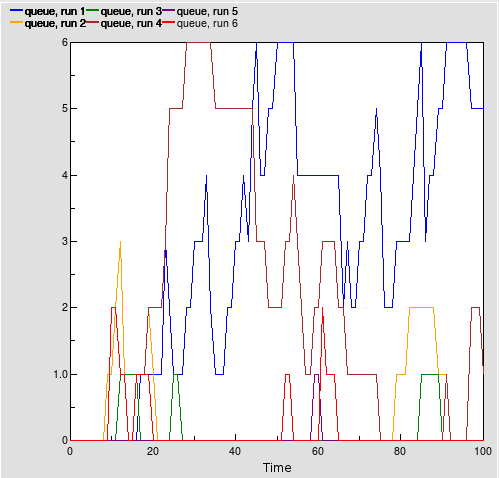## Queueing for a group of ATM machines

ModelId:
atm.sml
SimileVersion:
6.6p2

This model illustrates the use of Simile to create pure event-driven models. There are no compartments or explicit references to time in the model, so the state only changes when an event occurs. This means that the setting of the "Update every:" period has no effect on the model behaviour.

Category:
Technique
Equations:

Model Desktop1 :

Event   arrival : Customer arrives
arrival =         "true" (boolean)

This happens after a wait from the beginning of the simulation or from the last customer arrival

Event   next arrival : Wait for customer arrival
next arrival =         after(exprnd(4),"true") (boolean)

Customers arrive ar random intervals, so time between arrivals is given by the exponential distribution. Wait starts either at start of simulation or on arrival of previous customer.

Event   start : Start of simulation
start =         time() (boolean)
Maximum = 0
Provides an event at time 0, the start of the simulation.

State   queue : Number of customers waiting
queue =         [[arrival,prev(0)+(if full then 1 else 0)],[reset...,0],[machine/de-queue,prev(0)-1]] (int)
Where:
full = Value(s) of full

This starts at zero, increases by 1 if a customer arrives when all machines are full, and decreases by one when a customer leaves the queue

Variable   full : All machines are in use
full =         all([in_use]) (boolean)
Where:
[in_use] = Value(s) of machine/in use

Submodel machine :

Submodel "machine" is a fixed_membership multi-instance submodel with dimensions .

Event   de-queue : Customer moves from queue to machine
de-queue =         queue>0 (boolean)
Where:
queue = Value(s) of ../queue

Happens if someone finishes using a machine while people are waiting

Event   finish using : Customer completes transaction
finish using =         after(gaussian_var(10,1),"true") (boolean)

A period of time sampled from a normal distribution has elapsed since the customer started using the machine. Customer either started using machine which was idle, or came out of the queue.

Event   start using : Machine comes into use
start using =         not unreached and not in_use (boolean)
Where:
in_use = Value(s) of in use
unreached = Value(s) of unreached

Happens when this is the first free machine and a customer arrives and starts using it immediately

State   in use : Machine is busy.
in use =         [[finish using,queue>0],[start using,"true"],[reset...,"false"]] (boolean)
Where:
queue = Value(s) of ../queue

Machine becomes busy when a customer arrives and starts using it, and remains busy until a customer finishes using it while the queue is empty.

Variable   unreached : New customers do not reach this machine
unreached =         in_preceding(prev(0) or not in_use) (boolean)
Where:
in_use = Value(s) of in use